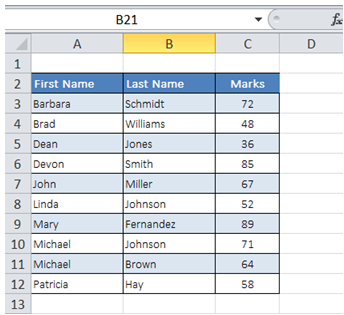# Excel Made Easy

## Vba find the last cell used in a column in Excel

For example, in the table below in "Sheet1" of my WorkBook, I would like to find the last cell in the First Name column.To do it in Excel, here is the answer:

1. Option Explicit
2. Sub LastRowLastColumn()
3. Dim lngLastRow As Long, lngLastColumn As Long
4. ''
5. lngLastRow = ThisWorkBook.Sheets("Sheet1").Cells(ThisWorkBook.Sheets("Sheet1").Rows.Count, 1).End(xlUp).Row
6. lngLastColumn = ThisWorkBook.Sheets("Sheet1").Cells(2, ThisWorkBook.Sheets("Sheet1").Columns.Count).End(xlToLeft).Column
7. ''
8. End Sub

Description:

a) In row 5, item marked in red represent the column no. (in this case First Name is in Col A, hence the value is one) where last used cell is to be determined.

b) Row 6 is used to determine last used cell in a row.

c) In row 6, item marked in red represent the row no. where last used cell is to be determined.